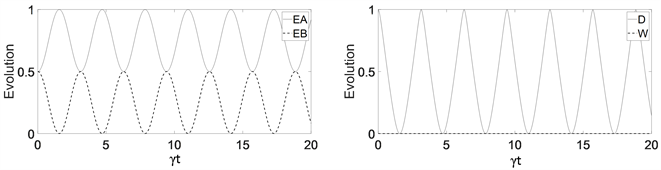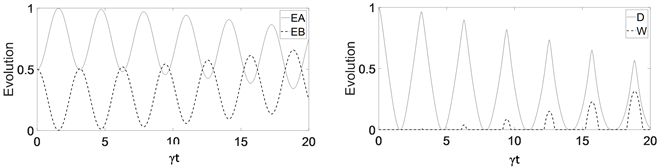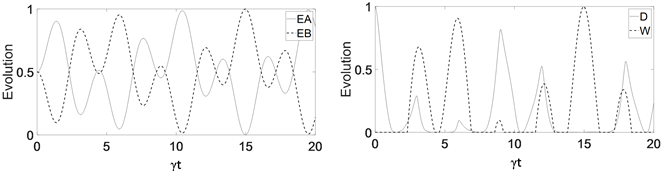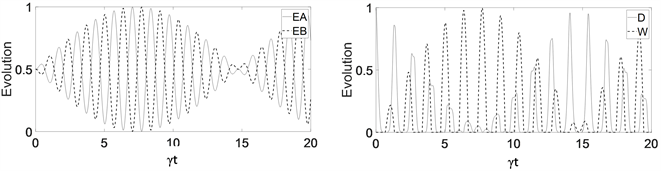#### 期刊菜单

The Role of Quantum Discord in Quantum Battery
DOI: 10.12677/MP.2021.116015, PDF, HTML, XML, 下载: 181  浏览: 397

Abstract: In this paper, we use a two-qubit system to simulate the quantum battery charger model. By accu-rately solving that two identical two-level atoms are in a single-mode cavity field system, the effects of quantum discord on energy conversion and ergotropy in the quantum battery under different average photon numbers are discussed. The results show that the quantum discord provides the power for the transmission of low energy to high energy in the quantum battery system, and the ergotropy will change with the increase of the number of photons. When the number of photons is small, the evolution of the system is greatly affected by quantum discord. With the increase of the number of photons, the weight of the number of photons in the evolution becomes larger and larger, resulting in a more complex evolution of the system.

1. 引言

2. 理论描述

2.1. 量子失协

$I\left(a:b\right)=H\left(a\right)+H\left(b\right)-H\left(a,b\right)$ (1)

$J\left(a,b\right)=H\left(a\right)-H\left(a|b\right)=H\left(b\right)-H\left(b|a\right)$ (2)

$I\left({\rho }_{ab}\right)=S\left({\rho }_{a}\right)+S\left({\rho }_{b}\right)-S\left({\rho }_{ab}\right)=S\left({\rho }_{a}\right)-S\left(a|b\right)$ (3)

$D\left({\rho }_{ab}\right)=I\left({\rho }_{ab}\right)-{C}^{\prime }\left({\rho }_{ab}\right)$ (4)

${C}^{\prime }\left({\rho }_{ab}\right)=S\left({\rho }_{a}\right)-\underset{\left\{{\Pi }_{k}\right\}}{\mathrm{min}}\left[S\left({\rho }_{ab}|\left\{{\Pi }_{k}\right\}\right)\right]$ (5)

$D\left({\rho }_{ab}\right)=S\left({\rho }_{b}\right)-S\left({\rho }_{ab}\right)+\underset{\left\{{\Pi }_{k}\right\}}{\mathrm{min}}S\left({\rho }_{ab}|\left\{{\Pi }_{k}\right\}\right)$ (6)

2.2. 理论模型

$H={H}_{0}+{H}_{1}$ (7)

${H}_{0}=\frac{1}{2}\omega {\sigma }_{z}^{A}+\frac{1}{2}\omega {\sigma }_{z}^{B}+\nu {a}^{+}a$ (8)

${H}_{1}=g{\mu }_{1}\left({a}^{+}{\sigma }_{-}^{A}+a{\sigma }_{+}^{A}\right)+g{\mu }_{2}\left({a}^{+}{\sigma }_{-}^{B}+a{\sigma }_{+}^{B}\right)$ (9)

$\frac{\text{d}\rho \left(t\right)}{\text{d}t}=-\frac{i}{\hslash }\left[{H}_{I},\rho \left(t\right)\right]$ (10)

$\rho \left(t\right)=U\rho \left(0\right){U}^{+}$ (11)

$\begin{array}{c}U=\mathrm{exp}\left(-i{H}_{I}t/\hslash \right)\\ =\mathrm{exp}\left[-i\lambda t\left(a{\sigma }_{+}^{A}+{a}^{+}{\sigma }_{-}^{A}\right)\right]\otimes \mathrm{exp}\left[-i\lambda t\left(a{\sigma }_{+}^{B}+{a}^{+}{\sigma }_{-}^{B}\right)\right]\\ ={U}_{A}\otimes {U}_{B}\end{array}$ (12)

${U}^{+}={U}_{B}^{+}\otimes {U}_{A}^{+}$ (13)

$\rho \left(t\right)=\left(\begin{array}{cccc}{\rho }_{11}& 0& 0& {\rho }_{14}\\ 0& {\rho }_{22}& {\rho }_{23}& 0\\ 0& {\rho }_{32}& {\rho }_{33}& 0\\ {\rho }_{41}& 0& 0& {\rho }_{44}\end{array}\right)$ (14)

$W\left(\tau \right)=Tr\left({\rho }_{B}\left(\tau \right){H}_{B}\right)-Tr\left({\sigma }_{\rho B}{H}_{B}\right)$ (15)

$W\left(\tau \right)={\omega }_{0}\left(2\left({\rho }_{11}+{\rho }_{33}\right)-1\right)\Theta \left(\left({\rho }_{11}+{\rho }_{33}\right)-\frac{1}{2}\right)$ (16)

3. 分析与讨论(a) (b)

Figure 1. Average photon number $n={10}^{-5}$ : (a) Evolution curve of charger energy (solid line) and battery energy (dotted line); (b) Evolution curves of quantum discord D (solid line) and ergotropy W (dotted line)(a) (b)

Figure 2. Average photon number $n={10}^{-3}$ : (a) Evolution curve of charger energy (solid line) and battery energy (dotted line); (b) Evolution curves of quantum discord D (solid line) and ergotropy W (dotted line)(a) (b)

Figure 3. Average photon number n = 0.1: (a) Evolution curve of charger energy (solid line) and battery energy (dotted line); (b) Evolution curves of quantum discord D (solid line) and ergotropy W (dotted line)(a) (b)

Figure 4. Average photon number $n=5$ : (a) Evolution curve of charger energy (solid line) and battery energy (dotted line); (b) Evolution curves of quantum discord D (solid line) and ergotropy W (dotted line)

4. 结论

NOTES

*通讯作者。

  Einstein, A., Podolsky, B. and Rosen, N. (1935) Can Quantum-Mechanical Description of Physical Reality Be Consi-dered Complete? Physical Review, 47, 696-702. https://doi.org/10.1103/PhysRev.47.777  Chang, P.Y., Chen, X., Gopalakrishnan, S., et al. (2019) Evolution of Entanglement Spectra under Generic Quantum Dynamics. Physical Review Letters, 123, Article No. 190602. https://doi.org/10.1103/PhysRevLett.123.190602  Modi, K., Brodutch, A., Cable, H., et al. (2011) The Classical-Quantum Boundary for Correlations: Discord and Related Measures. Reviews of Modern Physics, 84, 1655-1707. https://doi.org/10.1103/RevModPhys.84.1655  Ollivier, H. and Zurek, W.H. (2002) Quantum Discord: A Measure of the Quantumness of Correlations. Physical Review Letters, 88, Article No. 017901. https://doi.org/10.1103/PhysRevLett.88.017901  Brodutch, A. (2012) Discord and Quantum Computational Resources. Physical Review A, 88, 6893-6903. https://doi.org/10.1103/PhysRevA.88.022307  Datta, A., Shaji, A. and Caves, C.M. (2008) Quantum Discord and the Power of One Qubit. Physical Review Letters, 100, Article No. 050502. https://doi.org/10.1103/PhysRevLett.100.050502  Daki, B., Lipp, Y.O., Ma, X., et al. (2012) Quantum Discord as Resource for Remote State Preparation. Nature Physics, 8, 666-670. https://doi.org/10.1038/nphys2377  Giorgi, G.L. (2013) Quantum Discord and Remote State Preparation. Physical Review A, 88, 22315-22315. https://doi.org/10.1103/PhysRevA.88.022315  Su, X.L. (2014) Applying Gaussian Quantum Discord to Quantum Key Distribution. Chinese Science Bulletin, 59, 1083-1090. https://doi.org/10.1007/s11434-014-0193-x  Pirandola, S. (2014) Quantum Discord as a Resource for Quantum Cryptography. Scientific Reports, 4, Article No. 6956. https://doi.org/10.1038/srep06956  Grimaudo, R., Mihaescu, T., Isar, A., et al. (2019) Dynamics of Quantum Discord of Two Coupled Spin-1/2’s Subjected to Time-Dependent Magnetic Fields. Results in Physics, 13, Article No. 102147. https://doi.org/10.1016/j.rinp.2019.02.083  Campaioli, F., Pollock, F.A. and Vinjanampathy, S. (2018) Quantum Batteries. In: Binder, F., Correa, L., Gogolin C., Anders J. and Adesso G., Eds., Thermodynamics in the Quantum Regime, Springer, Cham. https://doi.org/10.1007/978-3-319-99046-0_8  Tabesh, F.T., Kamin, F.H. and Salimi, S. (2020) Environment-Mediated Charging Process of Quantum Batteries. Physical Review A, 102, Article No. 052223. https://doi.org/10.1103/PhysRevA.102.052223  Kamin, F.H., Tabesh, F.T., Salimi, S., et al. (2020) Non-Markovian Effects on Charging and Self-Discharging Process of Quantum Batteries. New Journal of Physics, 22, Article No. 083007. https://doi.org/10.1088/1367-2630/ab9ee2  Caravelli, F., Yan, B., Garcia-Pintos, L.P., et al. (2020) Energy Storage and Coherence in Closed and Open Quantum Batteries. Quantum, 5, 505. https://doi.org/10.22331/q-2021-07-15-505  Bai, M., Xu, H.J. and Yan, X.Q. (2020) Dynamics of the Quantum Discord with Weak Measurement for a Two-Atom System in Thermal Reservoirs. American Journal of Modern Physics, 9, 68-72. https://doi.org/10.11648/j.ajmp.20200905.11  Francica, G., Goold, J., Paternostro, M., et al. (2017) Daemonic Ergotropy: Enhanced Work Extraction from Quantum Correlations. npj Quantum Information, 3, Article No. 12. https://doi.org/10.1038/s41534-017-0012-8  Allahverdyan, A.E., Balian, R. and Nieuwenhuizen, T.M. (2002) Curie-Weiss Model of the Quantum Measurement Process. Europhysics Letters, 61, 452-458. https://doi.org/10.1209/epl/i2003-00150-y